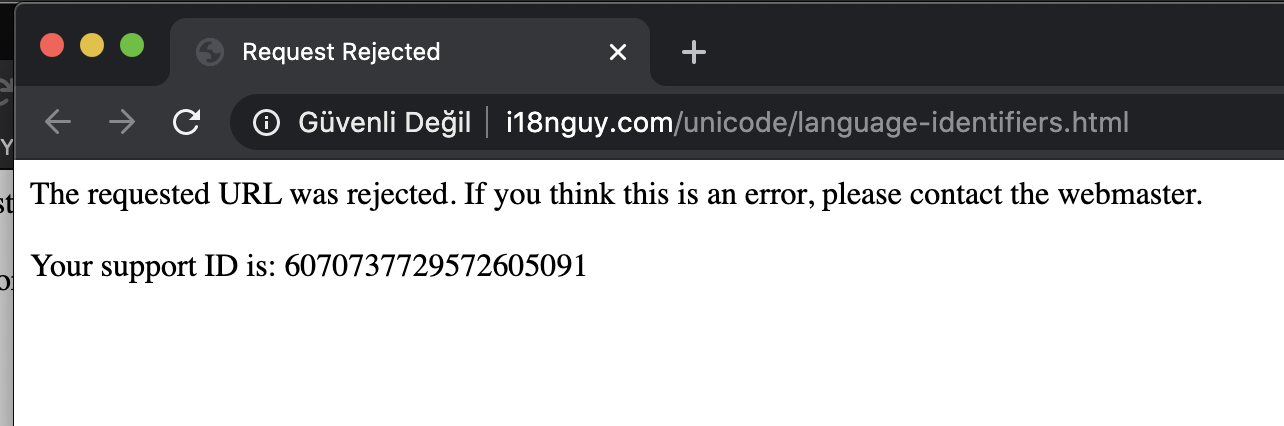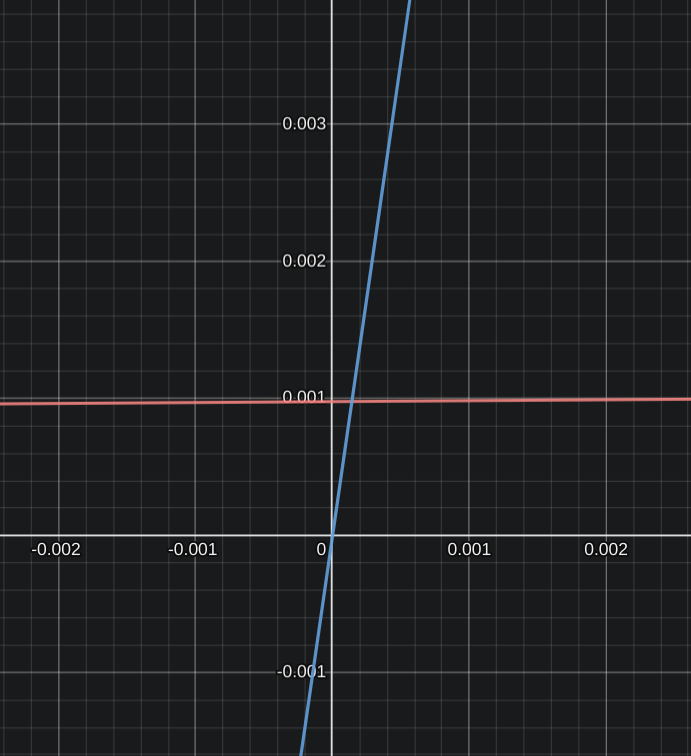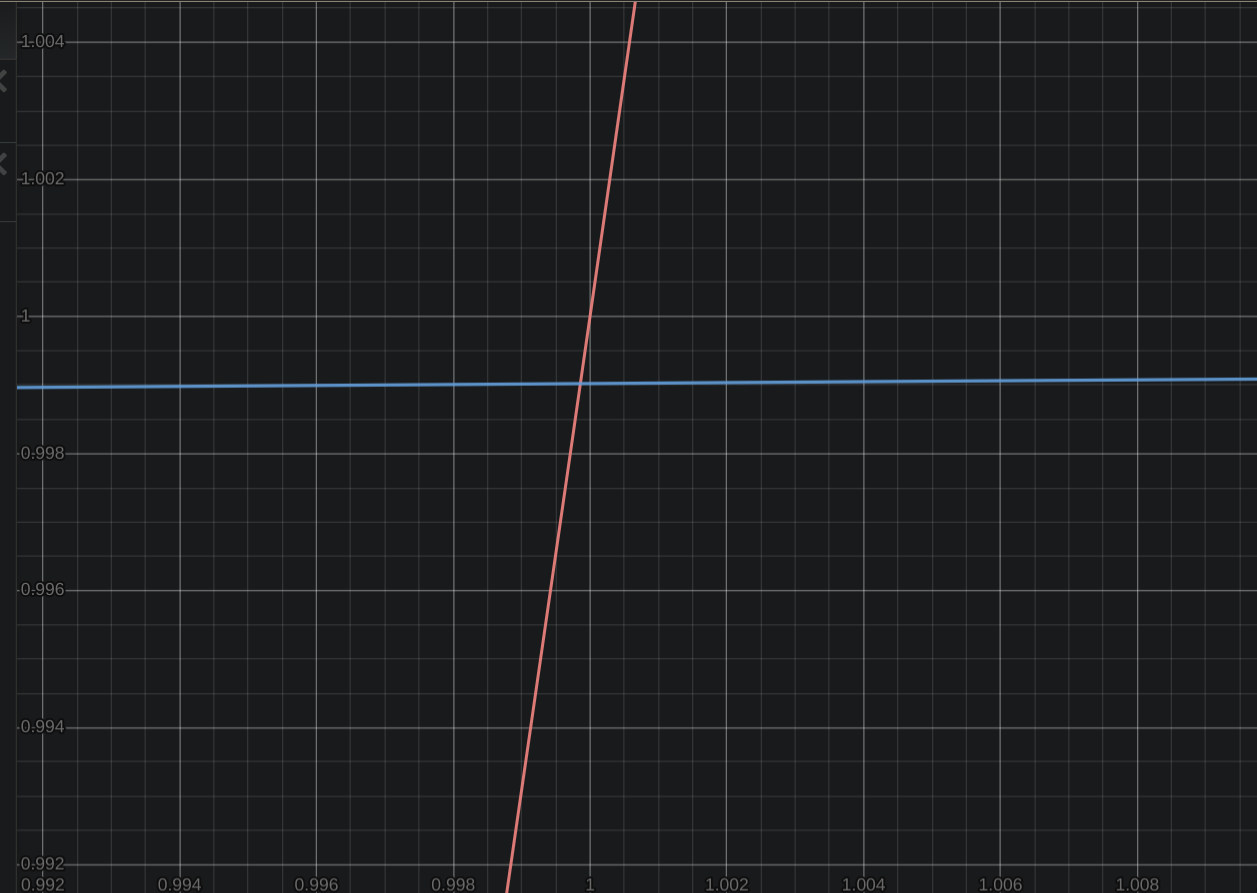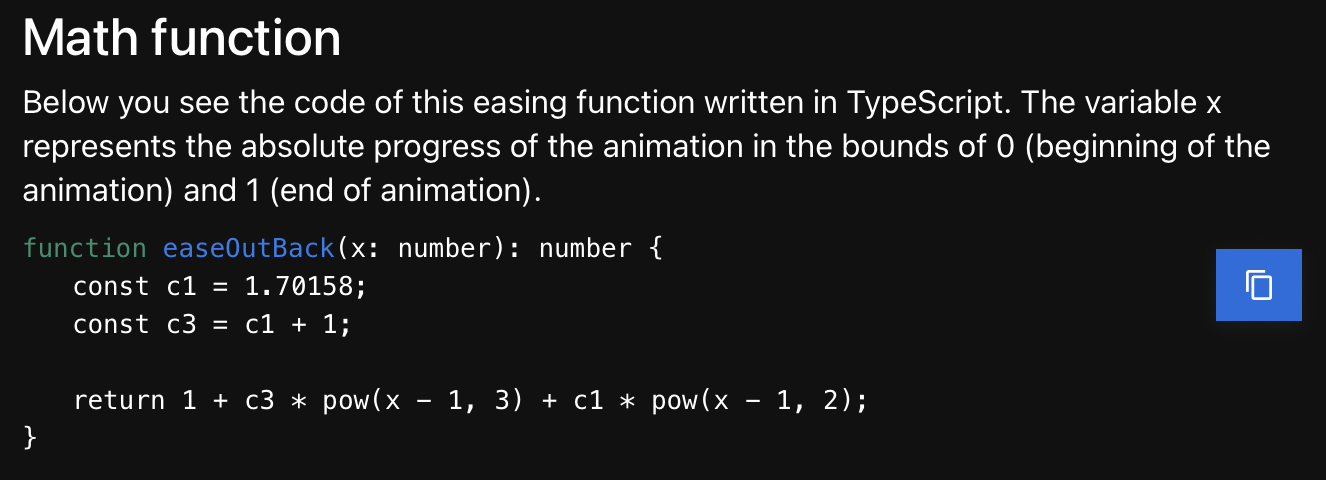# Easing Functions Cheat Sheet

• By Andrey Sitnik
• Last update: Jan 5, 2023

# Easing Functions Cheat Sheet

Simple cheat sheet to help developers pick the right easing function.## Contributing

GitHub has great instructions on how to set up Git, fork a project and make pull requests. If you have a problem with Git, just send your files directly to [email protected].

### Translate

Just copy the `i18n/en.yml` file to `i18n/CODE.yml` (where `CODE` is the lowercased RFC 3066 language code of your target language, for example `fr-ca` for Canadian French) and translate all messages.

### Test

1. Install project dependencies:

`yarn install`
2. That’s all. Run development server:

`yarn run start`
3. And open [localhost:1234] in browser.

## Github

https://github.com/ai/easings.net

• 1

### Перезапустить сайт

Что переделать:

• В примерах только CSS, PostCSS и CSS-в-JS.
• js-изинги переписать на `@keyframes`.
• После добавления новых строк, старые переводы станут неполные. Такие переводы надо будет выключить, но оставить в репозитории, чтобы переводчикам не надо было бы переводить с начала.
• Добавить своё имя в футер, но оставить моё.
• PWA со всеми иконками и манифестами.
• Поддержку офлайна и мгновенной загрузки.
• Переделать сборку с Ruby на JS.
• Автоматический деплой из ветки `master`.
• Версию справа-на-лево сделать через CSS Logical Properties.
• Поменять направление графиков в версии справа-на-лево.

Что оставить:

• Текущие адреса `/:locale` и `/:locale#:easing`
• Авторедирект исходя из языка браузера.
• Предложение перевести сайт, если языка пользователя нет.
• Возможность двойным кликом по заголовку выделить имя изинга.
• Маленький размер и простоту кода.
• Адаптивность и поддержку тач-интерфейса.
• Дизайн выделяющий содержимое, а не элементы интерфейса.
• Все графики на 13" мониторе должны быть видны без скролла.

Желательно:

• Загибающабщийся лепесток со ссылкой на исходники.
• Лучше, чтобы офлайн включалсь явно через заметный переключатель. Так о фиче будут знать.
• Может стоит сделать сразу ссылку на инструмент редактирования изингов, который сделала Лиа Веру?
• Добавить примеры для Android и iOS?
• 2

Victoria Tepfer sent me the translation to Hebrew

``````version: 1
lang_code: il
lang_name: עיברית
rtl: true

title: פונקציית הריכוך (Easing Functions)
description:
הפוך את האנימציה ליותר מציאותית על ידי בחירת פונקציית הריכוך הנכונה.
share:
פונקציית הריכוך (Easing Functions) קובע את מהירות של האנימציה, כך שהיא נראת יותר מציאותית
באתר הזה ניתן לראות איך אמורה להיראות עקומה, כך שאובייקט יזוז יותר טבעי וכמה שיותר קרוב למציאות

~~ פונקציית הריכוך (Easing Functions)~~ קובעת את מהירות תזוזה באנימצייה

דברים אמיתיים לא מתחילים לנוע באופן מיידי ובמהירות קבועה.
כשפותחים מגירה, ראשית אנו מאיצים אותה ואז מאיטים.
וכאשר הכדור נופל הוא מאיץ כל הזמן, ואחרי שפוגע ברצפה הוא קופץ מעט, עד שעוצר

האתר הזה יעזור לך למצוא את הפונקצייה שמתאימה לך

howtos:
css:
text: ב- CSS, מאפייני המעבר וההנפשה מאפשרים לך לציין פונקציית הקלה.
edit: לערוך ב ^cubic-bezier.com^.
with_animation: ב- CSS ניתן ליישם פונקציה זו עם {{@keyframes}}
example_size: גודל
example_position: עמדה
example_opacity: שקיפות

postcss:
text:
ב- PostCSS להישתמש בפונקצייה פשוט יותר.
יש פלגין ^postcss-easings^, שלוקח מידע מהאתר הזה.
explanation: הצהרה זו מומרת לזו שתוארה לעיל.
disabled:
למרבה הצער, לא ניתן להגדיר פונקציית החלקה זו באמצעות תוסף כלשהו PostCSS.
ניתן לעשות זאת עם {{@keyframes}}.

name: מדרון צבעים
text: אפשר לצייר שיפוע עם ^postcss-easing-gradients^.

mathfunction:
name: פונקציית מתמטיקה
text:
להלן מופיע הקוד לפונקציית המעבר שנכתב TypeScript.
משתנה X זו התקדמות של האנימציה, איפה ש 0 זה תחילת אנימציה ו 1 – זה סופה

easing:
all_easings: כל הפונקציות
check: לבדוק את השינוי של האטה
check_size: גודל
check_position: מיקום
check_opacity: שקיפות
current_func: פונקציה נוככית
linear_func: פונקציה לינארית

opensource:
title: Open Source
translate: Help translate site to your language

authors:
sitnik: Andrey Sitnik
separator: and
solovev: Ivan Solovev

theme:
auto: אוטומטי
light: בצבע בהיר
dark: צבע קהה
``````

The problem is that we need to create a right-to-left design (mirror design). You can try to use RTLCSS tool for that.

• 3

### Provide math equations

the visualization for easings is really useful, but needing to look up the equations how to implement them elsewhere is kind of missing the point.

It would be cool if there were code snippets for supported easings, for example under the images comparing linear with the easing function.

Usually I look at http://www.gizma.com/easing/ for reference, but I like the visualization of easings.net better. However because the code snippets on the other site are very easy to all see and copy, I usually go there instead.

Maybe it should be written more as pseudo-code with better variable names or just pure math equation though to be better understandable to everyone.

• 4

### Easy function copy

Hi,

I use your page a lot as a reference for CSS easings, they add more feel to just about any UI animation.

I find myself selecting and copying the blue `cubic-beizer(...)` text a lot... There are awesome buttons to preview the easing for different animations, would it be possible to add a small button that copies the CSS easing function to your clipboard?

For those that don't like installing CSS plugins that transform easings automatically, this website is godsent.

• 5

### better heading for jQuery / JavaScript

You were a bit too fast ;-)

Oversaw one line and I hope this is better than just jQuery as heading as there are Velocity and probably more that I do not know.

• 6

### Licence for the actual equations?

Hi. I needed a set of easing equations for an MIT licenced Unity project. I grabbed these and ported them to C# before I spotted that this was GPL 3.0

I did a quick Github search and I can find several MIT licenced repos that have the same equations with the same names so I'm hoping that your copyright isn't on the actual formulae and names themselves.

I can redo the port from another source but I'd rather not - so I thought I'd check with you.

The project that makes use of them is here: https://github.com/IxxyXR/Polyhydra-upm

and the ported code is here: https://github.com/IxxyXR/polyhydra-upm/blob/master/Packages/Polyhydra/com.ixxy.polyhydra/Conway/Runtime/Easing.cs

Happy to add copyright/credits or anything you request - I just don't want to change my licence to GPL. I'd rather redo the port from another source if that's required.

• 7

### Examples for Less

Hello! Could you add an example for Less? I created a repository. This repository contains the variables that are generated based on your project and an example for Less.

• 8

### GSAP easings and howto added

I have been using GSAP for a very long time now and I believe it really should be added to this project with the hope of helping others to do nicer animations.

I added all the easing names for GSAP, a description and an example to the main page and each easing type.

• 9

1. The Language code link in readme.md is using the insecure http protocol. Why are you giving external link? You can put the language codes markdown table in readme.md.webcache adress; http://webcache.googleusercontent.com/search?q=cache:L79OZ-daqVsJ:www.i18nguy.com/unicode/language-identifiers.html

• 10

### Real-world examples for different curves

Hey, I really like the idea behind easings.net. Thanks for the website.

I have an idea that maybe would be useful for the project. It would be great to have a short list of real life examples for each curve.

For example for easeOutQuad curve the simplest example is animation for removing an item from the list. Like this: https://stackoverflow.com/a/41223246/10558733 https://codepen.io/chriscoyier/pen/ozlJK

A list of links to examples hosted on codepen might works for this. What do you think?

• 11

### Equation cleanup

there are extra unused parameters and incorrect variable (re)declarations in some equations. The result is in fact inconsistant.

Here is a clean version (note: tabulations are set to 4 spaces, here GitHub uses 8 spaces):

``````// -----------------------------------------------------------
// -----------------------------------------------------------
jQuery.easing['jswing'] = jQuery.easing['swing'];
jQuery.extend(jQuery.easing, {
// unused:   x = null (for jQuery compatibility)
// required: t = current time, b = beginning value, c = change in value, d = duration
// optional: s = outer sweep for ease[In/Out]Back (default = 1.70158)
swing:            (x,t,b,c,d) =>
linear:           (x,t,b,c,d) =>
( t / d
) * c + b,
easeOutSine:      (x,t,b,c,d) =>
( Math.sin(t / d * Math.PI / 2)
) * c + b,
easeInSine:       (x,t,b,c,d) =>
( 1 - Math.cos(t / d * Math.PI / 2)
) * c + b,
easeInOutSine:    (x,t,b,c,d) =>
( (1 - Math.cos(t / d * Math.PI)) / 2
) * c + b,
( (t /= d) * (2 - t)
) * c + b,
( (t /= d) * t
) * c + b,
( (t /= d / 2) < 1
?         t *   t       / 2
: (1 - (--t) * (t - 2)) / 2
) * c + b,
easeOutCubic:     (x,t,b,c,d) =>
( (t = t/d - 1) * t * t + 1
) * c + b,
easeInCubic:      (x,t,b,c,d) =>
( (t /= d     ) * t * t
) * c + b,
easeInOutCubic:   (x,t,b,c,d) =>
( (t /= d / 2) < 1
?   t       * t * t      / 2
: ((t -= 2) * t * t + 2) / 2
) * c + b,
easeOutQuart:     (x,t,b,c,d) =>
( 1 - (t = (t = t / d - 1) * t) * t
) * c + b,
easeInQuart:      (x,t,b,c,d) =>
( (t = (t /= d) * t) * t
) * c + b,
easeInOutQuart:   (x,t,b,c,d) =>
(  (t /= d / 2) < 1
?     (t *=           t) * t / 2
: 1 - (t = (t -= 2) * t) * t / 2
) * c + b,
easeOutQuint:     (x,t,b,c,d) =>
( (t = t / d - 1) * (t *= t) * t + 1
) * c + b,
easeInQuint:      (x,t,b,c,d) =>
( (t /= d) * (t *= t) * t
) * c + b,
easeInOutQuint:   (x,t,b,c,d) =>
( (t /= d / 2) < 1
?       t       * (t *= t) * t / 2
: 1 + ((t -= 2) * (t *= t) * t / 2
) * c + b,
easeOutExpo:      (x,t,b,c,d) =>
( (t == d ? 0
: 1 - Math.pow(2, t / d * -10))
) * c + b,
easeInExpo:       (x,t,b,c,d) =>
( t == 0 ? 0
: Math.pow(2, (t / d - 1) * 10)
) * c + b,
easeInOutExpo:    (x,t,b,c,d) =>
( t == 0 ? 0 : (t /= d / 2) == 1 ? 1 : t < 1
?     Math.pow(2, (t - 1) * 10) / 2
: 1 - Math.pow(2, (1 - t) * 10) / 2
) * c + b,
easeOutCirc:      (x,t,b,c,d) =>
( Math.sqrt(1 - (t = t / d - 1) * t)
) * c + b,
easeInCirc:       (x,t,b,c,d) =>
( 1 - Math.sqrt(1 - (t /= d) * t)
) * c + b,
easeInOutCirc:    (x,t,b,c,d) =>
( (t /= d / 2) < 1
? (1 - Math.sqrt(1 - t        * t)    ) / 2
: (    Math.sqrt(1 - (t -= 2) * t) + 1) / 2
) * c + b,
easeOutElastic:   (x,t,b,c,d) =>
( (t /= d) == 1 ? 1
: 1 - Math.pow(2, t * -10) * Math.cos(t * Math.PI / 0.15)
) * c + b,
easeInElastic:    (x,t,b,c,d) =>
( (t /= d) == 0 ? 0 : t == 1 ? 1
: Math.pow(2, (t -= 1) *  10) * Math.cos(t * Math.PI / 0.15)
) * c + b,
easeInOutElastic: (x,t,b,c,d) =>
( t == 0 ? 0 : (t /= d / 2) == 2 ? 2 : t < 1
?     Math.pow(2, (t -= 1) *  10) * Math.cos(t * Math.PI / 0.225) / 2
: 1 - Math.pow(2, (t -= 1) * -10) * Math.cos(t * Math.PI / 0.225) / 2
) * c + b,
easeOutBounce:    (x,t,b,c,d) =>
( (t /= d) < 1   / 2.75 ? (t                 * 7.5625) * t
: t        < 2   / 2.75 ? (t -= 1.5   / 2.75 * 7.5625) * t + 0.75
: t        < 2.5 / 2.75 ? (t -= 2.25  / 2.75 * 7.5625) * t + 0.9375
:                         (t -= 2.625 / 2.75 * 7.5625) * t + 0.984375
) * c + b,
easeInBounce:     (x,t,b,c,d) =>
( 1 - jQuery.easing.easeOutBounce(x, 1 - t / d, 0, 1, 1)
) * c + b,
easeInOutBounce:  (x,t,b,c,d) =>
( (t /= d / 2) < 1
?     jQuery.easing.easeInBounce (x, t,     0, 1, 1) / 2
: 1 + jQuery.easing.easeOutBounce(x, t - 1, 0, 1, 1) / 2
) * c + b,
easeOutBack:      (x,t,b,c,d,s) =>
( ( ((s=(s==undefined?1.70158:s) + 1) * (t = t / d - 1) + s) * t + 1) * t
) * c + b,
easeInBack:       (x,t,b,c,d,s) =>
( (  (s=(s==undefined?1.70158:s) + 1) * (t /= d)        - s) * t      * t
) * c + b,
easeInOutBack:    (x,t,b,c,d,s) =>
( (t /= d / 2) < 1
? (  (s=(s==undefined?1.70158:s) * 1.525 + 1) *  t       - s) * t * t / 2
: (  (s=(s==undefined?1.70158:s) * 1.525 + 1) * (t -= 2) + s) * t * t / 2 + 1
) * c + b,
});
``````

you can note that all functions have to perform

• a predivision of t by d
• a final multiplication by c and addition of b
• x is never used

There are too many parameters in all "easing" functions (making them not so easy to use) ! This could be avoided by passing a single normalized parameter t in 0..1, (and optional parameter s) so that all basic ease functions are also returning normalized values in 0..1 (possibly with external sweep in the middle near the two ends, if the easing is "amortized" on both ends.

As well the two parameters b and c are not so convenient: practical use prefer using start and end values directly (where b = start, c = end-start) for describing animations.

Ideally, there should be a clean way to control the maximum sweeping, not jsut for ease[In/Out]Back, but also ease[In/Out]Elastic (which should then use the "s" parameter too.

In the current implementation of ease[In/Out]Back, there's an internal variable "s" but it is incorrectly used and finally voided, producing the same value as the simplified equation above where the specific value of internal variables s and p are fixed, so that the formula reduces to a "sin(x + pi/2)" equivalent to a simpler "-cos(x)" (as written above).

I wonder if this behavior was the use of the old parameter x (always passed as null and taking needless memory and stack). This is probably an old bad design of jQuery's easings (which originally only defines the "linear" and "swing" modes, "swing" being an alias for the OutQuad method (find for implementing very "reactive" UI buttons, but not for realistic animations, especially for scenes in 2D/3D emulating normal physics, including in games)... Only the linear and quadratic curves are really realistic, quadratic to emulate objects falling down due to gravity. The elastic and bounding moves is not well suited because of arbitrarily fixed parameters. Even the existing bouncing is not realistic because it uses an infinitely hard surface and has no segment of elastic deceleration near the bounding surface (thing about how balls are bouncing on a surface: they are deformed and the surface is also deformed, both elastically, or sometimes semi-elastically (e.g. on non-compacted natural ground where this leaves a hole, and where a part of the energy is absorbed and not entirely reflected even on hard surfaces, e.g. when bouncing on a sand surface, or on water, sometimes even entering within water; another part is dissipated as temperature without "moving" any fragment of the bouncing surface, except temporarily, and another part of the energy creating "waves" around the impact and absorbing a lot of the energy but not as significant temperature elevation).

Note that .animate(...) can be chained in a queue (jQuery creates and uses a default queue for each animatable object) using .animate(...).animate(...).

jQuery provides unnecessary callbacks for start and end events, which should be just inserted in the queue as an .animate(...) with duration=0. Animations in jQuery still required more work.

As well easings should be easier to do for 2D/3D animations using Bezier splines curves: instead of just using static values (min and max for t=0 and t=1 respectively, we should be able to link two easings for each coordinate in 2D, or three easings in 3D).

As well the easings above should have a way to specificy the parameters fixing the differential speed (negative or positive) at start and end; the functions above have a single fixed value for start and end speeds with limited values: 0, 1 or infinite (this is fixed at end only for easeIn* and easeOut*, and arbitratrily fixed at both start and end on the same value for easeInOut*, splitting the range arbitrarily in two mirrored parts exactly in the middle of time range)...

Animations using quadratic, cubic or elliptic beziers cannot be designed so simply with basic bezier control points or radii or focal points for elliptic curves given in their coordinate space: we have to predecompose the Bezier to create a parametric equation based on time and measuring the length along the Bezier or ellipitic curve to compute the final coordinate. So these easings are mostly fitted only for 1D effects.

Outside jQuery, in the CSS or SVG or OpenGL working groups, there are ongoing works on what is needed to describe a 2D or 3D scene: none of these easings are usable, except the "linear" one for moves along small arcs. Animations are then just basic designs made for 1D

• 12

### Missing Math in Typescript for easeOutCirc

I just integrated all the TypeScript functions into a class, and noticed that for `easeOutCirc` throws an error for `sqrt`, as it should be `Math.sqrt`. Super small issue, but might be nice to fix 😅

• 13

### Add more easing function from DOTween

Hi There are more easing type and easing function in DOTween. Like "Flash" easing type. It is an open source project. You can see the easing Code in this url: https://github.com/Demigiant/dotween/blob/90093a5c775ea22c9b3a2c828557f22a743c2589/_DOTween.Assembly/DOTween/Core/Easing/EaseManager.cs Thanks.

• 14

### Exponential functions not accurateease in exp is the red line. as you can see, the line does not actually cross 0,0.

With the (x === 0 ? 0 : ...) this would make a noticeable jump

Futhermore, ease out exp also has the issue on the other end.the blue line being ease out exp

• 15

`cubic-bezier(1/3, 0, 2/3, 1/3)` yields equivalently quadratic curve `y=x^2` as the TypeScript code in math function section explains.

how about to use corresponding `cubic-bezier(0.33, 0, 0.67, 0.33)` for easeInQuad? also `cubic-bezier(0.33, 0.67, 0.67, 1)` for easeOutQuad.

i hope this would help us.

• 16

### More permissive license for easing functions snippets?

This site is fantastic, and I'd love to use the easing function TS snippets in an MIT licensed project. Is there any chance that the function snippets (not the whole website) could be MIT, Apache, CC, or public domain?It's not hard to find permissively licensed implementations for all of these, but it'd be nice to just copy straight from this site.